Learn data science and machine learning by building real-world projects on Jovian

Jovian Lecture 5

## Classifying CIFAR10 images using a ResNet and Regularization techniques in PyTorch

#### Training an image classifier from scratch to over 90% accuracy in less than 5 minutes on a single GPU

##### Part 6 of "PyTorch: Zero to GANs"

This post is the sixth in a series of tutorials on building deep learning models with PyTorch, an open source neural networks library. Check out the full series:

This notebook is an extension to the tutorial Image Classification using CNNs in PyTorch, where we trained a deep convolutional neural network to classify images from the CIFAR10 dataset with around 75% accuracy. Here are some images from the dataset: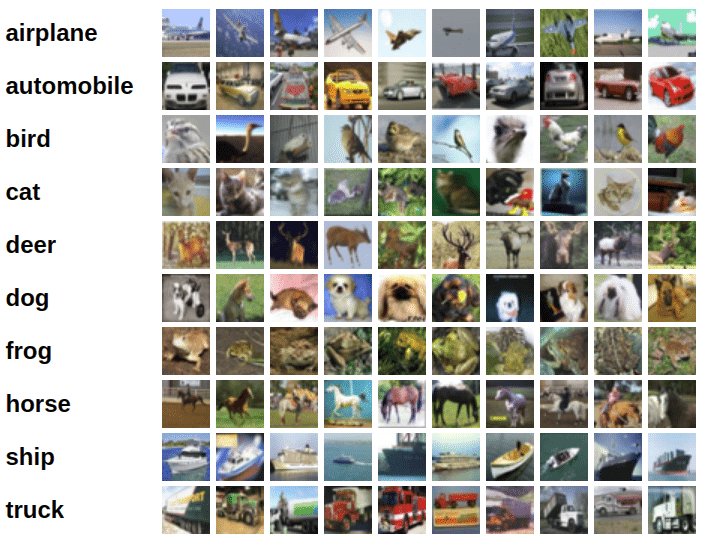In this tutorial, we'll use the following techniques to achieve over 90% accuracy in less than 5 minutes:

• Data normalization
• Data augmentation
• Residual connections
• Batch normalization
• Learning rate scheduling
• Weight Decay

### System Setup

This notebook is hosted on Jovian.ml, a platform for sharing data science projects. If you want to follow along and run the code as you read, you can choose the "Run on Kaggle" option from the "Run" dropdown above. Remember select "GPU" as the accelerator and turn on internet from the sidebar within the Kaggle notebook.

Otherwise, to run the code on your machine, you can clone the notebook, install the required dependencies using conda, and start Jupyter by running the following commands:

``````pip install jovian --upgrade               # Install the jovian library
cd 05b-cifar10-resnet                      # Enter the created directory
conda create -n 05b-cifar10-resnet         # Create virtual env
conda activate 05b-cifar10-resnet          # Activate virtual env
conda install jupyter                      # Install Jupyter
jupyter notebook                           # Start Jupyter
``````

For a more detailed explanation of the above steps, check out the System setup section in the first notebook. Before you start executing the code below, you may want to clear the cell outputs by selecting "Kernel > Restart and Clear Output" from the Jupyter notebook menu bar, to avoid confusion.

We begin by importing the required modules & libraries.

In :
``````# Uncomment and run the commands below if imports fail
# !conda install numpy pandas pytorch torchvision cpuonly -c pytorch -y
# !pip install matplotlib --upgrade --quiet``````
In :
``````import os
import torch
import torchvision
import tarfile
import torch.nn as nn
import numpy as np
import torch.nn.functional as F
from torchvision.datasets import ImageFolder
import torchvision.transforms as tt
from torch.utils.data import random_split
from torchvision.utils import make_grid
import matplotlib.pyplot as plt
%matplotlib inline
``````
In :
``project_name='05b-cifar10-resnixk'``

### Preparing the Data

In :
``````# Dowload the dataset
dataset_url = "http://files.fast.ai/data/cifar10.tgz"

# Extract from archive
with tarfile.open('./cifar10.tgz', 'r:gz') as tar:
tar.extractall(path='./data')

# Look into the data directory
data_dir = './data/cifar10'
print(os.listdir(data_dir))
classes = os.listdir(data_dir + "/train")
print(classes)
``````
```Downloading http://files.fast.ai/data/cifar10.tgz to ./cifar10.tgz ```
``HBox(children=(FloatProgress(value=1.0, bar_style='info', max=1.0), HTML(value='')))``
``` ['train', 'labels.txt', 'test'] ['deer', 'automobile', 'truck', 'frog', 'airplane', 'ship', 'cat', 'horse', 'dog', 'bird'] ```

There are a few important changes we'll make while creating the PyTorch datasets:

1. Use test set for validation: Instead of setting aside a fraction (e.g. 10%) of the data from the training set for validation, we'll simply use the test set as our validation set. This just gives a little more data to train with. In general, once you have picked the best model architecture & hypeparameters using a fixed validation set, it is a good idea to retrain the same model on the entire dataset just to give it a small final boost in performance.
2. Channel-wise data normalization: We will normalize the image tensors by subtracting the mean and dividing by the standard deviation across each channel. As a result, the mean of the data across each channel is 0, and standard deviation is 1. Normalizing the data prevents the values from any one channel from disproportionately affecting the losses and gradients while training, simply by having a higher or wider range of values that others.
3. Randomized data augmentations: We will apply randomly chosen transformations while loading images from the training dataset. Specifically, we will pad each image by 4 pixels, and then take a random crop of size 32 x 32 pixels, and then flip the image horizontally with a 50% probability. Since the transformation will be applied randomly and dynamically each time a particular image is loaded, the model sees slightly different images in each epoch of training, which allows it generalize better.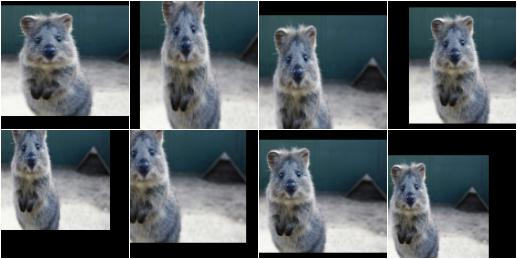In :
``````# Data transforms (normalization & data augmentation)
stats = ((0.4914, 0.4822, 0.4465), (0.2023, 0.1994, 0.2010))
tt.RandomHorizontalFlip(),
tt.ToTensor(),
tt.Normalize(*stats,inplace=True)])
valid_tfms = tt.Compose([tt.ToTensor(), tt.Normalize(*stats)])
``````
In :
``````# PyTorch datasets
train_ds = ImageFolder(data_dir+'/train', train_tfms)
valid_ds = ImageFolder(data_dir+'/test', valid_tfms)``````

Next, we can create data loaders for retrieving images in batches. We'll use a relatively large batch size of 500 to utlize a larger portion of the GPU RAM. You can try reducing the batch size & restarting the kernel if you face an "out of memory" error.

In :
``batch_size = 400``
In :
``````# PyTorch data loaders
train_dl = DataLoader(train_ds, batch_size, shuffle=True, num_workers=3, pin_memory=True)
valid_dl = DataLoader(valid_ds, batch_size*2, num_workers=3, pin_memory=True)``````

Let's take a look at some sample images from the training dataloader.

In :
``````def show_batch(dl):
for images, labels in dl:
fig, ax = plt.subplots(figsize=(12, 12))
ax.set_xticks([]); ax.set_yticks([])
ax.imshow(make_grid(images[:16], nrow=8).permute(1, 2, 0))
break``````
In :
``show_batch(train_dl)``The colors seem out of place because of the normalization. Note that normalization is also applied during inference. If you look closely, you can see the cropping and reflection padding in some of the images. Horizontal flip is a bit difficult to detect from visual inspection.

### Using a GPU

To seamlessly use a GPU, if one is available, we define a couple of helper functions (`get_default_device` & `to_device`) and a helper class `DeviceDataLoader` to move our model & data to the GPU as required. These are described in more detail in a previous tutorial.

In :
``````def get_default_device():
"""Pick GPU if available, else CPU"""
if torch.cuda.is_available():
else:

def to_device(data, device):
"""Move tensor(s) to chosen device"""
if isinstance(data, (list,tuple)):
return [to_device(x, device) for x in data]
return data.to(device, non_blocking=True)

"""Wrap a dataloader to move data to a device"""
def __init__(self, dl, device):
self.dl = dl
self.device = device

def __iter__(self):
"""Yield a batch of data after moving it to device"""
for b in self.dl:
yield to_device(b, self.device)

def __len__(self):
"""Number of batches"""
return len(self.dl)``````

Based on where you're running this notebook, your default device could be a CPU (`torch.device('cpu')`) or a GPU (`torch.device('cuda')`)

In :
``````device = get_default_device()
device
``````
Out:
``device(type='cuda')``

We can now wrap our training and validation data loaders using `DeviceDataLoader` for automatically transferring batches of data to the GPU (if available).

In :
``````train_dl = DeviceDataLoader(train_dl, device)
``````

### Model with Residual Blocks and Batch Normalization

One of the key changes to our CNN model this time is the addition of the resudial block, which adds the original input back to the output feature map obtained by passing the input through one or more convolutional layers.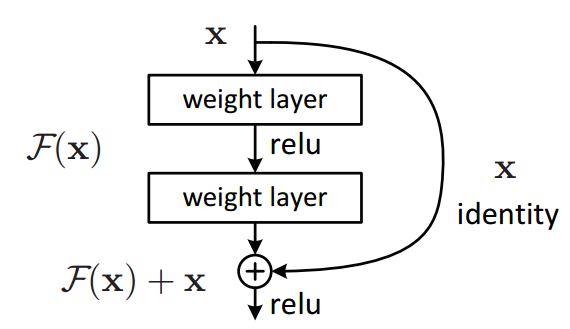Here is a very simply Residual block:

In :
``````class SimpleResidualBlock(nn.Module):
def __init__(self):
super().__init__()
self.conv1 = nn.Conv2d(in_channels=3, out_channels=3, kernel_size=3, stride=1, padding=1)
self.relu1 = nn.ReLU()
self.conv2 = nn.Conv2d(in_channels=3, out_channels=3, kernel_size=3, stride=1, padding=1)
self.relu2 = nn.ReLU()

def forward(self, x):
out = self.conv1(x)
out = self.relu1(out)
out = self.conv2(out)
return self.relu2(out) + x # ReLU can be applied before or after adding the input``````
In :
``````simple_resnet = to_device(SimpleResidualBlock(), device)

for images, labels in train_dl:
out = simple_resnet(images)
print(out.shape)
break

del simple_resnet, images, labels
torch.cuda.empty_cache()
``````
```torch.Size([400, 3, 32, 32]) ```

This seeming small change produces a drastic improvement in the performance of the model. Also, after each convolutional layer, we'll add a batch normalization layer, which normalizes the outputs of the previous layer.

We will use the ResNet9 architecture, as described in this blog series :In :
``````def accuracy(outputs, labels):
_, preds = torch.max(outputs, dim=1)

class ImageClassificationBase(nn.Module):
def training_step(self, batch):
images, labels = batch
out = self(images)                  # Generate predictions
loss = F.cross_entropy(out, labels) # Calculate loss
return loss

def validation_step(self, batch):
images, labels = batch
out = self(images)                    # Generate predictions
loss = F.cross_entropy(out, labels)   # Calculate loss
acc = accuracy(out, labels)           # Calculate accuracy
return {'val_loss': loss.detach(), 'val_acc': acc}

def validation_epoch_end(self, outputs):
batch_losses = [x['val_loss'] for x in outputs]
epoch_loss = torch.stack(batch_losses).mean()   # Combine losses
batch_accs = [x['val_acc'] for x in outputs]
epoch_acc = torch.stack(batch_accs).mean()      # Combine accuracies
return {'val_loss': epoch_loss.item(), 'val_acc': epoch_acc.item()}

def epoch_end(self, epoch, result):
print("Epoch [{}], last_lr: {:.5f}, train_loss: {:.4f}, val_loss: {:.4f}, val_acc: {:.4f}".format(
epoch, result['lrs'][-1], result['train_loss'], result['val_loss'], result['val_acc']))``````
In :
``````def conv_block(in_channels, out_channels, pool=False):
layers = [nn.Conv2d(in_channels, out_channels, kernel_size=3, padding=1),
nn.BatchNorm2d(out_channels),
nn.ReLU(inplace=True)]
if pool: layers.append(nn.MaxPool2d(2))
return nn.Sequential(*layers)

class ResNet9(ImageClassificationBase):
def __init__(self, in_channels, num_classes):
super().__init__()

self.conv1 = conv_block(in_channels, 64)
self.conv2 = conv_block(64, 128, pool=True)
self.res1 = nn.Sequential(conv_block(128, 128), conv_block(128, 128))

self.conv3 = conv_block(128, 256, pool=True)
self.conv4 = conv_block(256, 512, pool=True)
self.res2 = nn.Sequential(conv_block(512, 512), conv_block(512, 512))

self.classifier = nn.Sequential(nn.MaxPool2d(4),
nn.Flatten(),
nn.Linear(512, num_classes))

def forward(self, xb):
out = self.conv1(xb)
out = self.conv2(out)
out = self.res1(out) + out
out = self.conv3(out)
out = self.conv4(out)
out = self.res2(out) + out
out = self.classifier(out)
return out``````
In :
``````model = to_device(ResNet9(3, 10), device)
model
``````
Out:
``````ResNet9(
(conv1): Sequential(
(0): Conv2d(3, 64, kernel_size=(3, 3), stride=(1, 1), padding=(1, 1))
(1): BatchNorm2d(64, eps=1e-05, momentum=0.1, affine=True, track_running_stats=True)
(2): ReLU(inplace=True)
)
(conv2): Sequential(
(0): Conv2d(64, 128, kernel_size=(3, 3), stride=(1, 1), padding=(1, 1))
(1): BatchNorm2d(128, eps=1e-05, momentum=0.1, affine=True, track_running_stats=True)
(2): ReLU(inplace=True)
(3): MaxPool2d(kernel_size=2, stride=2, padding=0, dilation=1, ceil_mode=False)
)
(res1): Sequential(
(0): Sequential(
(0): Conv2d(128, 128, kernel_size=(3, 3), stride=(1, 1), padding=(1, 1))
(1): BatchNorm2d(128, eps=1e-05, momentum=0.1, affine=True, track_running_stats=True)
(2): ReLU(inplace=True)
)
(1): Sequential(
(0): Conv2d(128, 128, kernel_size=(3, 3), stride=(1, 1), padding=(1, 1))
(1): BatchNorm2d(128, eps=1e-05, momentum=0.1, affine=True, track_running_stats=True)
(2): ReLU(inplace=True)
)
)
(conv3): Sequential(
(0): Conv2d(128, 256, kernel_size=(3, 3), stride=(1, 1), padding=(1, 1))
(1): BatchNorm2d(256, eps=1e-05, momentum=0.1, affine=True, track_running_stats=True)
(2): ReLU(inplace=True)
(3): MaxPool2d(kernel_size=2, stride=2, padding=0, dilation=1, ceil_mode=False)
)
(conv4): Sequential(
(0): Conv2d(256, 512, kernel_size=(3, 3), stride=(1, 1), padding=(1, 1))
(1): BatchNorm2d(512, eps=1e-05, momentum=0.1, affine=True, track_running_stats=True)
(2): ReLU(inplace=True)
(3): MaxPool2d(kernel_size=2, stride=2, padding=0, dilation=1, ceil_mode=False)
)
(res2): Sequential(
(0): Sequential(
(0): Conv2d(512, 512, kernel_size=(3, 3), stride=(1, 1), padding=(1, 1))
(1): BatchNorm2d(512, eps=1e-05, momentum=0.1, affine=True, track_running_stats=True)
(2): ReLU(inplace=True)
)
(1): Sequential(
(0): Conv2d(512, 512, kernel_size=(3, 3), stride=(1, 1), padding=(1, 1))
(1): BatchNorm2d(512, eps=1e-05, momentum=0.1, affine=True, track_running_stats=True)
(2): ReLU(inplace=True)
)
)
(classifier): Sequential(
(0): MaxPool2d(kernel_size=4, stride=4, padding=0, dilation=1, ceil_mode=False)
(1): Flatten()
(2): Linear(in_features=512, out_features=10, bias=True)
)
)``````

### Training the model

Before we train the model, we're going to make a bunch of small but important improvements to our `fit` function:

• Learning rate scheduling: Instead of using a fixed learning rate, we will use a learning rate scheduler, which will change the learning rate after every batch of training. There are many strategies for varying the learning rate during training, and the one we'll use is called the "One Cycle Learning Rate Policy", which involves starting with a low learning rate, gradually increasing it batch-by-batch to a high learning rate for about 30% of epochs, then gradually decreasing it to a very low value for the remaining epochs. Learn more: https://sgugger.github.io/the-1cycle-policy.html

• Weight decay: We also use weight decay, which is yet another regularization technique which prevents the weights from becoming too large by adding an additional term to the loss function.Learn more: https://towardsdatascience.com/this-thing-called-weight-decay-a7cd4bcfccab

• Gradient clipping: Apart from the layer weights and outputs, it also helpful to limit the values of gradients to a small range to prevent undesirable changes in parameters due to large gradient values. This simple yet effective technique is called gradient clipping. Learn more: https://towardsdatascience.com/what-is-gradient-clipping-b8e815cdfb48

Let's define a `fit_one_cycle` function to incorporate these changes. We'll also record the learning rate used for each batch.

In :
``````@torch.no_grad()
model.eval()
outputs = [model.validation_step(batch) for batch in val_loader]
return model.validation_epoch_end(outputs)

def get_lr(optimizer):
for param_group in optimizer.param_groups:
return param_group['lr']

torch.cuda.empty_cache()
history = []

# Set up cutom optimizer with weight decay
optimizer = opt_func(model.parameters(), max_lr, weight_decay=weight_decay)
# Set up one-cycle learning rate scheduler
sched = torch.optim.lr_scheduler.OneCycleLR(optimizer, max_lr, epochs=epochs,

for epoch in range(epochs):
# Training Phase
model.train()
train_losses = []
lrs = []
loss = model.training_step(batch)
train_losses.append(loss)
loss.backward()

optimizer.step()

# Record & update learning rate
lrs.append(get_lr(optimizer))
sched.step()

# Validation phase
result['train_loss'] = torch.stack(train_losses).mean().item()
result['lrs'] = lrs
model.epoch_end(epoch, result)
history.append(result)
return history``````
In :
``````history = [evaluate(model, valid_dl)]
history
``````
Out:
``[{'val_loss': 2.3145909309387207, 'val_acc': 0.09605769068002701}]``

In :
``````epochs = 8
max_lr = 0.01
weight_decay = 1e-4
``````
In :
``````%%time
history += fit_one_cycle(epochs, max_lr, model, train_dl, valid_dl,
weight_decay=weight_decay,
opt_func=opt_func)
``````
```Epoch , last_lr: 0.00393, train_loss: 0.6951, val_loss: 0.7997, val_acc: 0.7259 Epoch , last_lr: 0.00935, train_loss: 0.8714, val_loss: 1.1328, val_acc: 0.6446 Epoch , last_lr: 0.00972, train_loss: 0.7496, val_loss: 0.7805, val_acc: 0.7459 Epoch , last_lr: 0.00812, train_loss: 0.6015, val_loss: 0.6191, val_acc: 0.7940 Epoch , last_lr: 0.00556, train_loss: 0.4718, val_loss: 0.4389, val_acc: 0.8485 Epoch , last_lr: 0.00283, train_loss: 0.3840, val_loss: 0.3754, val_acc: 0.8708 Epoch , last_lr: 0.00077, train_loss: 0.2674, val_loss: 0.3034, val_acc: 0.8958 Epoch , last_lr: 0.00000, train_loss: 0.1919, val_loss: 0.2681, val_acc: 0.9095 CPU times: user 42.6 s, sys: 8.54 s, total: 51.1 s Wall time: 4min 27s ```
In :
``train_time='4:07'``

Our model trained to over 90% accuracy in just 5 minutes! Try playing around with the data augmentations, network architecture & hyperparameters to achive the following results:

1. 94% accuracy in under 10 minutes (easy)
2. 90% accuracy in under 2.5 minutes (intermediate)
3. 94% accuracy in under 5 minutes (hard)

Let's plot the valdation set accuracies to study how the model improves over time.

In :
``````def plot_accuracies(history):
accuracies = [x['val_acc'] for x in history]
plt.plot(accuracies, '-x')
plt.xlabel('epoch')
plt.ylabel('accuracy')
plt.title('Accuracy vs. No. of epochs');``````
In :
``plot_accuracies(history)``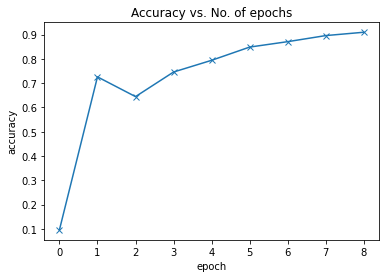We can also plot the training and validation losses to study the trend.

In :
``````def plot_losses(history):
train_losses = [x.get('train_loss') for x in history]
val_losses = [x['val_loss'] for x in history]
plt.plot(train_losses, '-bx')
plt.plot(val_losses, '-rx')
plt.xlabel('epoch')
plt.ylabel('loss')
plt.legend(['Training', 'Validation'])
plt.title('Loss vs. No. of epochs');``````
In :
``plot_losses(history)``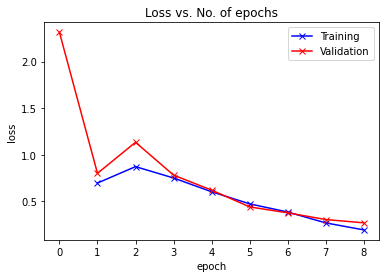It's clear from the trend that our model isn't overfitting to the training data just yet. Finally, let's visualize how the learning rate changed over time, batch-by-batch over all the epochs.

In :
``````def plot_lrs(history):
lrs = np.concatenate([x.get('lrs', []) for x in history])
plt.plot(lrs)
plt.xlabel('Batch no.')
plt.ylabel('Learning rate')
plt.title('Learning Rate vs. Batch no.');``````
In :
``plot_lrs(history)``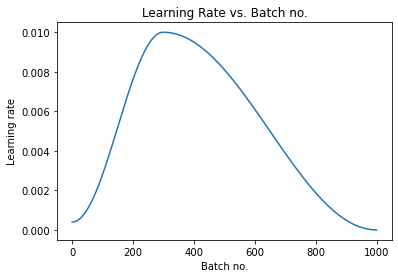As expected, the learning rate starts at a low value, and gradually increases for 30% of the iterations to a maximum value of `0.01`, and then gradually decreases to a very small value.

### Save and Commit

Let's save the weights of the model, record the hyperparameters, and commit our experiment to Jovian. As you try different ideas, make sure to record every experiment so you can look back and analyze the results.

In :
``torch.save(model.state_dict(), 'cifar10-resnet9.pth')``
In :
``!pip install jovian --upgrade --quiet``
In :
``import jovian``
In :
``````jovian.reset()
jovian.log_hyperparams(arch='resnet9',
epochs=epochs,
lr=max_lr,
scheduler='one-cycle',
weight_decay=weight_decay,
opt=opt_func.__name__)
``````
```[jovian] Please enter your API key ( from https://jovian.ml/ ): API KEY: ········ [jovian] Hyperparams logged. ```
In :
``````jovian.log_metrics(val_loss=history[-1]['val_loss'],
val_acc=history[-1]['val_acc'],
train_loss=history[-1]['train_loss'],
time=train_time)
``````
```[jovian] Metrics logged. ```
In :
``jovian.commit(project=project_name, environment=None, outputs=['cifar10-resnet9.pth'])``
```[jovian] Attempting to save notebook.. [jovian] Detected Kaggle notebook... [jovian] Uploading notebook to https://jovian.ml/nixkjadhav007/05b-cifar10-resnixk ```

Here's a summary of the different techniques used in this tutorial to improve our model performance and reduce the training time:

• Data normalization: We normalized the image tensors by subtracting the mean and dividing by the standard deviation of pixels across each channel. Normalizing the data prevents the pixel values from any one channel from disproportionately affecting the losses and gradients. Learn more

• Data augmentation: We applied random transformations while loading images from the training dataset. Specifically, we will pad each image by 4 pixels, and then take a random crop of size 32 x 32 pixels, and then flip the image horizontally with a 50% probability. Learn more

• Residual connections: One of the key changes to our CNN model was the addition of the resudial block, which adds the original input back to the output feature map obtained by passing the input through one or more convolutional layers. We used the ResNet9 architecture Learn more.

• Batch normalization: After each convolutional layer, we added a batch normalization layer, which normalizes the outputs of the previous layer. This is somewhat similar to data normalization, except it's applied to the outputs of a layer, and the mean and standard deviation are learned parameters. Learn more

• Learning rate scheduling: Instead of using a fixed learning rate, we will use a learning rate scheduler, which will change the learning rate after every batch of training. There are many strategies for varying the learning rate during training, and we used the "One Cycle Learning Rate Policy". Learn more

• Weight Decay: We added weight decay to the optimizer, yet another regularization technique which prevents the weights from becoming too large by adding an additional term to the loss function. Learn more

`` ``